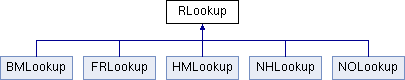RLookup Class Reference

Inheritance diagram for RLookup:List of all members.

Public Member Functions

virtual RLookup_Types WhatType () const =0
virtual void Populate (RoutingVec_t &r, RoutingVec_t &p, nodeid_t d=NODE_NONE, nodeid_t o=NODE_NONE, nodeid_t f=NODE_NONE, nodeid_t l=NODE_NONE)=0
virtual void Populate (istream &is)
virtual nodeid_t Lookup (nodeid_t)=0
virtual size_t Size ()=0
virtual size_t NumberEntries ()
virtual void Log (ostream &)

Static Public Member Functions

static void Analyze (RoutingVec_t &, RoutingVec_t &, nodeid_t &, nodeid_t &, nodeid_t, nodeid_t &, nodeid_t &)

The documentation for this class was generated from the following file:
• /Users/yzchen/ns/ns-allinone-2.33/ns-2.33/routealgo/rlookup.h